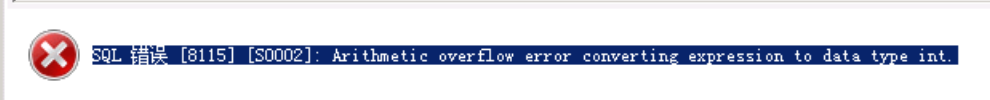# SQL Server Error: Arithmetic overflow error converting expression to data type int.

1. Problem description

SQL Server (SQL DW) queries the number of data in a table and reports an error using count

``````select count(*)  from test.test_t;
``````

Then an error is reported:

``````SQL ERROR  [S0002]: Arithmetic overflow error converting expression to data type int.
``````2. Cause of the problem

The amount of data is relatively large. The query result directly with count is of type int, which exceeds the range of int.

tinyint: integer from 0 to 255
smallint: integer from – 2 15 (-32768) to 2 15 (32767)
int: integer from – 2 31 (-2147483648) to 2 31 (2147483647)
bigint: integer data (all numbers) from -2 63 (-9223372036854775808) to 2 63 -1 (9223372036854775807) decimal: numeric data with fixed precision and range
from -10 38 -1 to 10 38 -1

3. Solution

Microsoft sql provides count_big method to count

``````select count_big(*)  from test.test_t;
``````